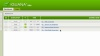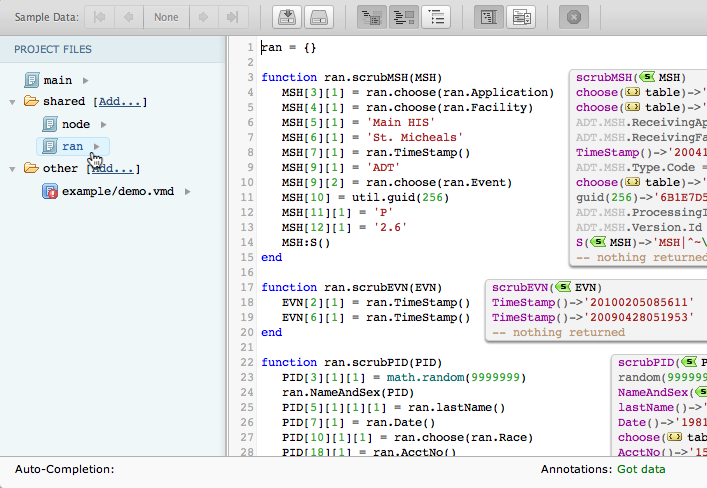# The Iguana Demo

## Contents

This simple channel produces random HL7 ADT messages and places them into an external folder for the ’01-File to Socket’ channel to pick up and process. We added this channel to ensure that there is a constant stream of HL7 data running through your demo channels while they are active.## How It Works

The 04-Test Data Generator demo channel consists of the following components, each designed to perform a specific task:

Component Type Function
Source ‘From Translator'(script) Polls every 10000 ms (or 10 seconds) to produce a random ADT message and push it to the queue
Filter inactive n/a
Destination ‘To File’ Converts messages to .txt format and places them in an external folder using unique file names for each: iNTERFACEWARE/Iguana/Demo/Messages/

Every channels includes a Filter component; however, since we don’t need any filtering to perform this task, we have left it inactive (default).

## Understanding the Code

If you are having difficulty understanding this channel’s code, don’t panic. This section explains each line of the script to help you get better oriented.

```require 'node'
require 'ran'```

Our first step is to import all the shared modules that we will need:

• The ‘node’ module converts a node to a string (and escape any characters that need escaped)
• The ‘ran’ module generates the ADT messages
`function main()`

The `main()` function is the primary function that Iguana calls when running a script. The rest of your code simply defines the tasks that `main()` is in charge of executing.

`queue.push{data=ran.RandomMessage()}`

This line represents the only instructions we need. It triggers the ‘ran’ module and then pushes the results back into the queue.

This channel’s main script may seem pretty short, but there is a lot of code working under the hood. By putting all that code into its own reusable module (‘ran’), we make sharing and duplicating this task extremely easy. Now you don’t have to write the scripts out by hand; you can simply call the module in your `main()` function.

### About the ‘ran’ module

Let’s walk through the ‘ran’ module to give you a better idea of how we generate random HL7 messages. To access this code, simply click on the module’s hyperlink in the Project Files panel:`ran = {}`

This line creates a blank table that we will use to store our functions.

```function ran.scrubMSH(MSH)
MSH = ran.choose(ran.Application)
MSH = ran.choose(ran.Facility)
MSH = 'Main HIS'
MSH = 'St. Micheals'
MSH = ran.TimeStamp()
MSH = ran.choose(ran.Event)
MSH = util.guid(256)
MSH = 'P'
MSH = '2.6'
MSH:S()
end```

This function creates the MSH segment.

```function ran.scrubEVN(EVN)
EVN = ran.TimeStamp()
EVN = ran.TimeStamp()
end```

This function creates the EVN segment by running the ‘TimeStamp’ function (defined below).

```function ran.scrubPID(PID)
PID = math.random(9999999)
ran.NameAndSex(PID)
PID = ran.lastName()
PID = ran.Date()
PID = ran.choose(ran.Race)
PID = ran.AcctNo()
PID, PID = ran.location()
PID = math.random(99999)
PID = math.random(999)..
' '..ran.choose(ran.Street)
PID = ran.SSN()
PID:S()
end```

This function creates the PID segment.

```function ran.PV1(PV1)
PV1 = ran.lastName()
PV1 = ran.firstName()
PV1 = 'F'
PV1 = math.random(9999999)
PV1 = ran.TimeStamp()
PV1:S()
end```

This function creates the PV1 segment.

```function ran.NK1(NK1)
for i = 1, math.random(6) do
NK1[i] = i
ran.Kin(NK1[i])
end
end```

This function uses the math.random() to create a random number of NK1 segment(s).

```function ran.Kin(NK1)
NK1 = ran.lastName()
NK1 = ran.firstName()
NK1 = ran.choose(ran.Relation)
end```

This function creates each unique next of kin records to populate the NK1 segments.

```ran.Sex = {'M', 'F'}
ran.MaleNames = {'Fred','Jim','Gary','John'} ran.FemaleNames = {'Mary','Sabrina','Tracy'}
ran.Race = {'AI', 'EU', 'Mixed', 'Martian', 'Unknown'} ran.Street = {'Delphi Cres.', 'Miller Lane', 'Yonge St.', 'Main Rd.'} ran.Relation = {'Grandchild', 'Second Cousin', 'Sibling', 'Parent'} ran.Event = {'A01', 'A03', 'A04', 'A05', 'A06', 'A07', 'A08'} ran.Facility = {'Lab', 'E&R'} ran.Application = { 'AcmeMed', 'MedPoke', 'CowZing' }
ran.Locations = { {'Chicago', 'IL'}, {'Toronto', 'ON'}, {'ST. LOUIS', 'MO'}, {'LA', 'CA'} }```

These tables store the options that we randomly choose for the outgoing ADT message. You can add additional choices if you need to produce more HL7 transactions, or if you want alter their properties.

```function ran.Date()
local T = os.date('*t')
local newDate = '19'..rand(T.year,99,2)..
rand(T.month,12,2)..
rand(T.day,29,2)
return newDate
end
function rand(In, Max, Size)
local Result = tostring((In + math.random(Max)) % Max)
if '0' == Result then
Result = '1'
end
while Size > Result:len() do
Result = '0'..Result
end
return Result
end```

These functions are used to randomly choose a date and time.